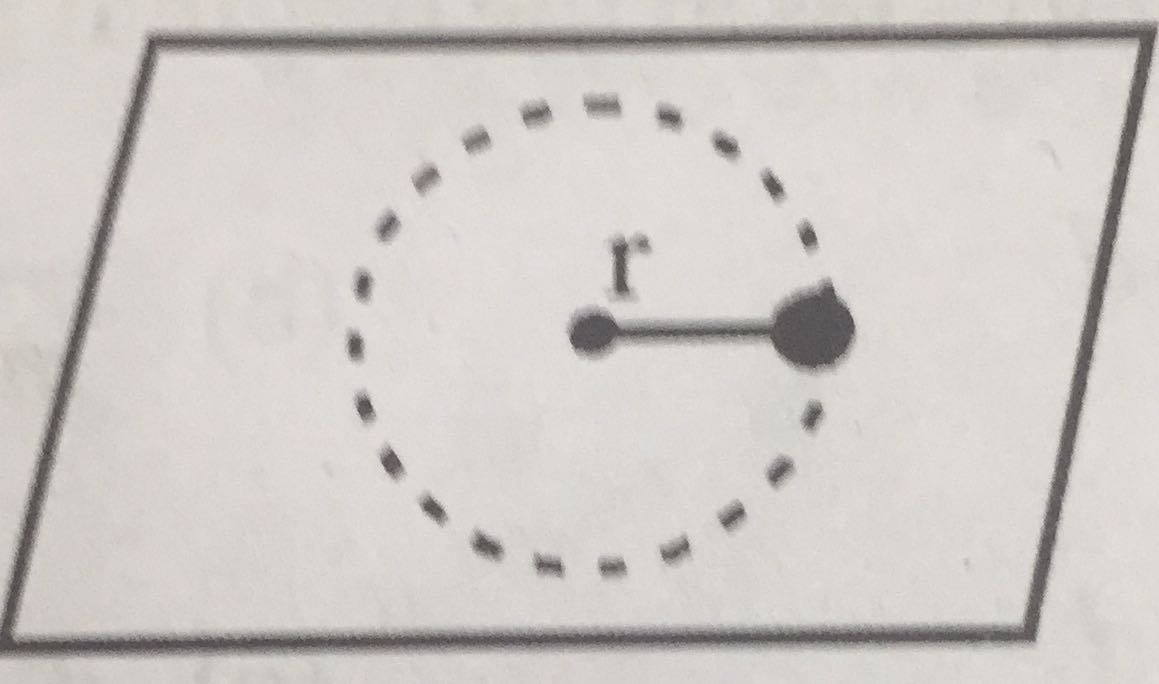## Filters

Sort by :
Clear All
Q
Medical
309 Views   |

A small mass attached to a string rotates on frictionless table top as shown. If the tension in the string is increased by pulling the string causing the radius of the cutcular motion to decrease by a factor of 2, the kinetic eneegy of the mass will• Option 1)

remain constant

• Option 2)

increase by a factor of 2

• Option 3)

increase by a factor of 4

• Option 4)

decrease by a factor of 2

As discussed in Angular momentum - - wherein   represent angular momentum of a moving particle about a point. it can be calculated  as = Length of perpendicular on line of motion = component of momentum along perpendicualar to   is increased by a factor of 4  Option 1) remain constant This option is incorrect. Option 2) increase by a factor of 2 This option is incorrect. Option...
Exams
Articles
Questions